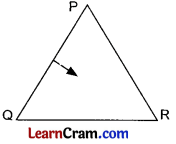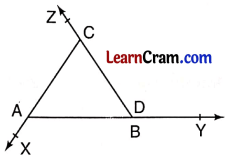# DAV Class 6 Maths Chapter 12 Worksheet 2 Solutions

The DAV Maths Book Class 6 Solutions and DAV Class 6 Maths Chapter 12 Worksheet 2 Solutions of Triangles offer comprehensive answers to textbook questions.

## DAV Class 6 Maths Ch 12 WS 2 Solutions

Question 1.
In the given triangle, name the:(a) Vertex opposite to side QR.
(b) Side opposite to vertex Question
(c) Side opposite to vertex R.
(d) Vertex opposite to side PQuestion
(a) P
(b) PR
(c) PQ
(d) R

Question 2.
Draw any triangle ABC and mark the points:
(a) X, Y in the interior of the ΔABC.
(b) R, S in the exterior of the ΔABC.
(a) In ΔABC, X, Y are in the interior of ΔABC.
(b) R and S are in the exterior of ΔABC.Question 3.
What do you mean by triangular region?
The region in the interior of the triangle and its sides is called its triangular region.

Question 4.
Look carefully at the following figure and name:(a) all the triangles formed.
(b) the triangles which have the point P in its exterior.
(c) the triangles which have the point P in its interior.
(b) ΔAOD, ΔDOC, ΔBOC, ΔADC, ΔBDC
(c) ΔAOB, ΔABD, ΔABC

Question 5.
In the given figure, name the triangles which have:(a) A as one vertex (any 2)
(b) B as one vertex (any 2)
(c) E as one vertex (any 2) id) DF as one side (any 2)
(e) BC as one side
(f) AE as one side.